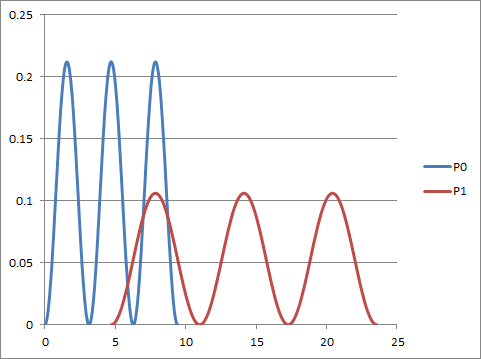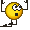# How to transform a probability density function?

• A

TL;DR Summary
Transforming a trimodal probability density function with support [0;3*Pi] to a trimodal probability density function with support [(3/2)*Pi;(15/2)*Pi] .
I have the following probability density function (in Maple notation):

f (x) = (1 / ((3/2) * Pi)) * (sin (x)) ** 2 with support [0; 3 * Pi]

Now I want to transform x so that

0 -> (3/2) * Pi
and
3 * Pi -> (15/2) * Pi

and the new function is still a probability density function.

How should I do that transformation?

Hi,
0 -> (3/2) * Pi
and
3 * Pi -> (15/2) * Pi
It is a simple linear transformation.
So if we define ##x_0=0,\ x_1 = {3\over 2}\pi,\quad x_0'= 3\pi,\ x_1'={15\over2}\pi \;,\ ## then: $$x'= {x-x_0\over x_1-x_0} (x_1'-x'_0) \ \ {\sf and} \ \ dx'= {x'_1-x'_0\over x_1-x_0}\ dx$$

So you substitute ##\displaystyle {x = {x' - x_0'\over x_1'-x_0'}(x_1-x_0)}## and

with a simple factor ##{dx/ dx'}## you preserve the integral.•Dale

I have performed the transformation and I got (in Maple notation):

g (x) = (1/2) * (1 / ((3/2) * Pi)) * (sin ((1/2) * (x- (3/2) * Pi))) ** 2

with support [(3/2) * Pi; (15/2) * Pi)]

but this function coincides completely with the following function:

h (x) = (1 / (6 * Pi)) * (sin (x) +1)

also with support [(3/2) * Pi; (15/2) * Pi)]

Now g (x) is a quadratic function and h (x) is not. How can they coincide?

sin2(x) = 1/2*(1 - cos(2x))

Thank you very much.